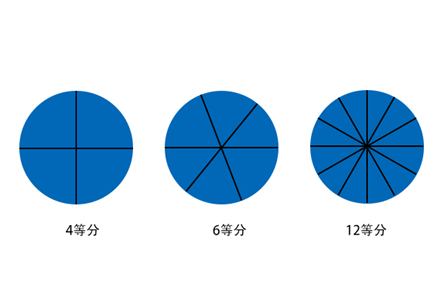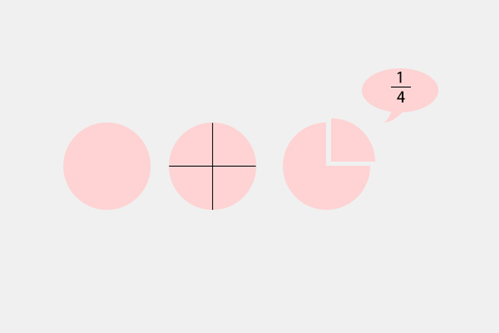2021-09-22 15:39:52      来源：新华网1=1/3+1/5+1/7+1/9+1/11+1/15+1/35+1/45+1/231

1=1/3+1/5+1/7+1/9+1/11+1/15+1/21+1/135+1/10395

1=1/3+1/5+1/7+1/9+1/11+1/15+1/21+1/165+1/693

1=1/3+1/5+1/7+1/9+1/11+1/15+1/21+1/231+1/315

1=1/3+1/5+1/7+1/9+1/11+1/15+1/33+1/45+1/385

24小时网站违法和不良信息举报电话:0871-65390101  举报邮箱:2779967946＠qq.com